How Cheenta works to ensure student success?
Explore the Back-Story

# Cyclic Groups & Subgroups : IIT 2018 Problem 1# Understand the problem

Which one of the following is TRUE? (A)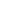is cyclic if and only if n is prime
(B) Every proper subgroup ofis cyclic
(C) Every proper subgroup of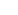is cyclic
(D) If every proper subgroup of a group is cyclic, then the group is cyclic.

Hint 1:

We will solve this question by the method of elimination. Observe that if n is prime thenis obviously cyclic as any of the subgroup <a> has order either 1 or n by Lagrange's theorem. Now if the order is 1 then a=id. So choose a(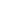e)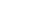then |<a>|=n and <a>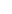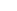<a>=. The problem will occur with the converse see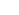is cyclic but 6 is not prime. In general= <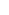> is always cyclic no matter what n is!! so option (A) is false. Can you rule out option (C)

Hint 2:

Consider option (C) every proper subgroup ofis cyclic. Consider { e , (12)(34) , (13)(24) , (14)(23) } = G  Observe that this is a subgroup and |G|=4. Moreover o(g)=2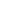g(e)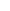G So G is not cyclic. Hence option (C) is not correct. Can you rule out option (D)?

Hint 3:

Consider*which is also known as Klein's 4 group then it is not cyclic but all of it's proper subgroups are {0}*,*{0} and {0}*{0} which are cyclic. Hence we can rule out option (D) as well.

Hint 4:

So option (B) is correct. Now let prove that H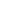= {,,.....,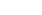}. By well ordering principle H has a minimal non zero element 'm'. Claim: H=<m> clearly <m>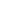H. For any rH by Euclid's algorithm we have r=km+d where 0d < m  whichd=r-kmH If d0 then d<m which is a contradiction So, d=0r=kmH=<m> and we are done

# Connected Program at Cheenta

The higher mathematics program caters to advanced college and university students. It is useful for I.S.I. M.Math Entrance, GRE Math Subject Test, TIFR Ph.D. Entrance, I.I.T. JAM. The program is problem driven. We work with candidates who have a deep love for mathematics. This program is also useful for adults continuing who wish to rediscover the world of mathematics.

# Understand the problem

Which one of the following is TRUE? (A)is cyclic if and only if n is prime
(B) Every proper subgroup ofis cyclic
(C) Every proper subgroup ofis cyclic
(D) If every proper subgroup of a group is cyclic, then the group is cyclic.

Hint 1:

We will solve this question by the method of elimination. Observe that if n is prime thenis obviously cyclic as any of the subgroup <a> has order either 1 or n by Lagrange's theorem. Now if the order is 1 then a=id. So choose a(e)then |<a>|=n and <a><a>=. The problem will occur with the converse seeis cyclic but 6 is not prime. In general= <> is always cyclic no matter what n is!! so option (A) is false. Can you rule out option (C)

Hint 2:

Consider option (C) every proper subgroup ofis cyclic. Consider { e , (12)(34) , (13)(24) , (14)(23) } = G  Observe that this is a subgroup and |G|=4. Moreover o(g)=2g(e)G So G is not cyclic. Hence option (C) is not correct. Can you rule out option (D)?

Hint 3:

Consider*which is also known as Klein's 4 group then it is not cyclic but all of it's proper subgroups are {0}*,*{0} and {0}*{0} which are cyclic. Hence we can rule out option (D) as well.

Hint 4:

So option (B) is correct. Now let prove that H= {,,.....,}. By well ordering principle H has a minimal non zero element 'm'. Claim: H=<m> clearly <m>H. For any rH by Euclid's algorithm we have r=km+d where 0d < m  whichd=r-kmH If d0 then d<m which is a contradiction So, d=0r=kmH=<m> and we are done

# Connected Program at Cheenta

The higher mathematics program caters to advanced college and university students. It is useful for I.S.I. M.Math Entrance, GRE Math Subject Test, TIFR Ph.D. Entrance, I.I.T. JAM. The program is problem driven. We work with candidates who have a deep love for mathematics. This program is also useful for adults continuing who wish to rediscover the world of mathematics.

# Similar Problems

This site uses Akismet to reduce spam. Learn how your comment data is processed.

### Knowledge Partner